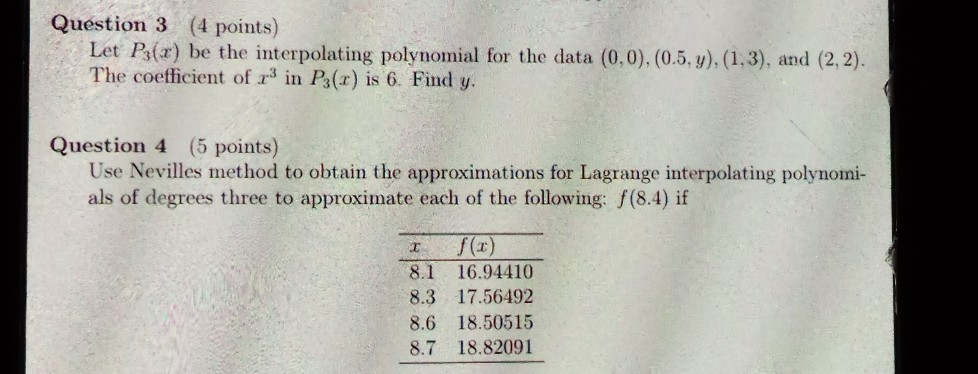# Question Question 3 (4 points) Let P3(r) be the interpolating polynomial for the data (0,0), (0.5,y),(1,3), and (2,2). The coefficient of 23 in P3(x) is 6. Find y. Question 4 (5 points) Use Nevilles method to obtain the approximations for Lagrange interpolating polynomi- als of degrees three to approximate each of the following: f(8.4) if І 8.1 16.94410 8.3 17.56492 8.6 18.50515 8.7 18.82091